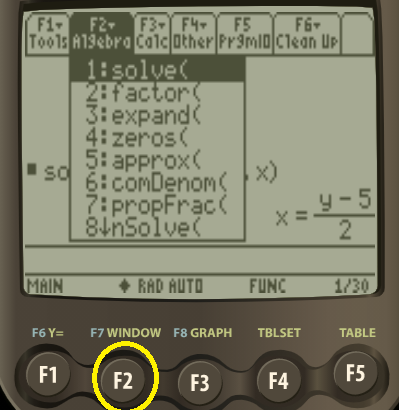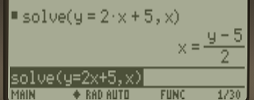# Express x as a Function of y

## What Does “Express x as a Function of y” Mean?

When we say “Express x as a Function of y”, we mean to manipulate a function so that you can feed y into it and get x. In other words, you want to rearrange a formula so that x is on the left.

A fairly easy way to remember what you should be solving for is that you are working from left to right in the formula exactly in exactly the same order as the wording in the problem. For example:

 y as a function of x x as a function of y y = x – 1 x = y + 1 y = 2x + 9 x = (y – 9) /2

In beginning calculus, you’re usually working with y as a function of x (in other words, you feed x in, and get out y). Once you start working with volumes and double integrals, you’ll sometimes need to work in terms of other variables.

## Example

Example question: Express x as a function of y:
y = 2x – 5.

Solution
The function is written in terms of y as a function of x. We want to get this in terms of x as a function of y (i.e. we want to switch the function around so that x is on the left). We must do a little algebra to get “x” on its own:

1. Switch sides: 2x – 5 = y
2. Add 5 to both sides: 2x – 5 + 5 = y + 5 → 2x = y + 5
3. Divide both sides by 2: (2x)/2 = (y + 5)/2 → x = (y + 5)/2

Caution: This technique is sometimes referred to as “inverting a function” but it is not the same as finding an inverse function. when you find an inverse function, you’re still keeping the same variable on the same side. For example, the inverse of y = 2x – 5 is y = (x – 5)/2.

## Using a Calculator to Express x as a Function of y

You can express x as a function of y on the TI-89 calculator. Here’s how to solve the above question using the TI-89:

Step 1: Press the F2 button.Step 2: Press 1 for “solve”.

Step 3: Type in your function, followed by a comma, followed by x.

Step 4: Press Enter to get the solution.If you don’t own a TI-89 calculator, they have a great online TI-89 simulator here.

You can also use Symbolab’s “solve for” calculator to solve any equation for x. Make sure to type in your variable (x) in this case and then a comma, before typing in the equation you want to switch variables for:CITE THIS AS:
Stephanie Glen. "Express x as a Function of y" From StatisticsHowTo.com: Elementary Statistics for the rest of us! https://www.statisticshowto.com/express-x-as-a-function-of-y/